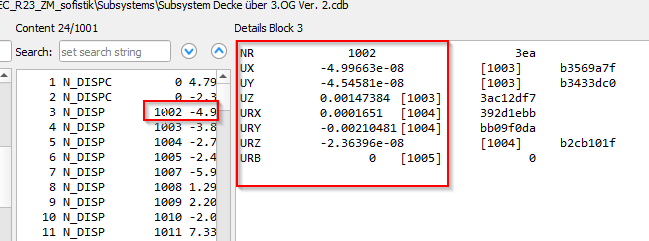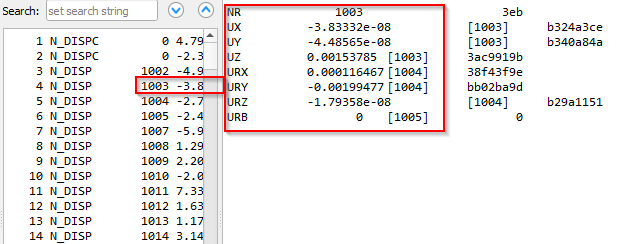# Discrepency in extraction of 'nodal support Z' from CDB

Hello Everyone,

For nearly a year, I’ve been using Python interfaces to extract information from CDB. However, this time, while extracting the `nodal support Z` from the CDB, I encountered a discrepancy. It seems that some nodes, which should have no ‘pz’ value in the CDB, are returning unexpected or repetitive values. I’m currently unable to determine where these values are coming from.

As you can see in the figures below:

• Figure 1: Indicates that the node did not have any ‘pz’ value.
• Figure 2: However, when the same node is extracted using Python, it returns a value.

I’m seeking assistance in understanding this inconsistency and finding a resolution. Any insights or guidance would be greatly appreciated.

Thank you.

Fig-1Fig-2

Code:

``````def get_forces(Index, LC):
forces = []
ie = c_int(0)
while ie.value < 2:
datalen = sizeof(CN_DISPC)
RecLen = c_int(sizeof(cn_dispc))
ie.value = py_sof_cdb_get(Index, 24, LC, byref(cn_dispc), byref(RecLen), 1)
if cn_dispc.m_id >0:
forces.append({
'NR': cn_dispc.m_id,
'LF': LC,
'P-Z': round(cn_dispc.m_pz,2)

})
RecLen = c_int(sizeof(cn_dispc))
return forces
``````

@sfr or @JFH someone could help me

Hard to say without a system to play around with.
I normally use the result viewer for reaction forces and export to excel.

The cdb records/structs (like 24/LC) usually all have the same data length.

Since you have junk data it’s:

• Either from variables being uninitialised
• Or from reading the cdb

Try setting the values to zero before reading.
If that helps you were dealing with an uninitialised variable. In that case you might need to clear the values between each read.

If that doesn’t work you either need to cross reference the nodes that actually have supports.
Another option is to read forces from support elements (springs if you got them).

1 Like

Thanks, @sfr. I attempted initializing the struct with zero values. I’m not sure whether you were referring to the struct variables or Python storage variables. However, initializing it to zero proved to be effective in resolving the issue. I implemented it using the following code.
Please comment on it if this is the correct approach for initializing and reinitializing the struct variable to zero, or there will be potential issues (due to misshandling of variable) in the long run.

``````def get_forces(Index, LC):
forces = []
ie = c_int(0)

# Initialize the struct variable to zero
cn_dispc = CN_DISPC()
cn_dispc.m_id = 0
cn_dispc.m_pz = 0.0

while ie.value < 2:
datalen = sizeof(CN_DISPC)
RecLen = c_int(sizeof(cn_dispc))
ie.value = py_sof_cdb_get(Index, 24, LC, byref(cn_dispc), byref(RecLen), 1)
if cn_dispc.m_id > 0 and cn_dispc.m_pz:
forces.append({
'NR': cn_dispc.m_id,
'LF': LC,
'P-Z': round(cn_dispc.m_pz, 2)
})
# Reinitialize the struct and relevant fields to zero
cn_dispc.m_id = 0
cn_dispc.m_pz = 0.0
RecLen = c_int(sizeof(cn_dispc))
return forces
``````

Thanks again, @sfr.